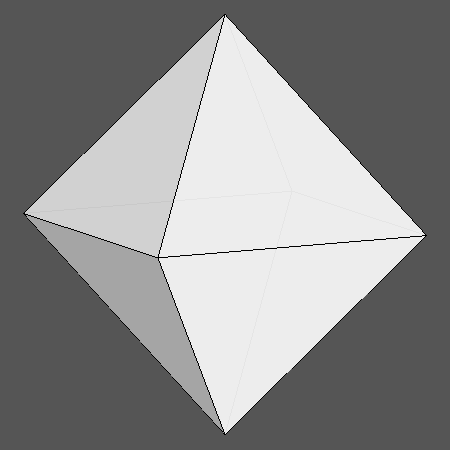Acronym ... Name cubically truncated rhombic dodecahedron,chamfered octahedron©© Vertex figure [3,H2], [h4] General of army (is itself convex) Colonel of regiment (is itself locally convex) Dihedral angles (at margins) between {3} and {(h,H,H)2}:   arccos[-sqrt(2/3)] = 144.735610° between {(h,H,H)2} and {(h,H,H)2}:   120° Confer rad   oct ExternallinksThe non-regular hexahedra {(h,H,H)2} have vertex angles h = arccos(1/3) = 70.528779° resp. H = arccos[-sqrt(2/3)] = 144.735610°.

Cubical truncation applies to the cubical vertices (vertex inscribed cube) only. Wrt. the rad this produces new triangle faces there. These are then face planes of a cutting oct. – The above transition shows a dynamical mutual scaling of oct and rad. The chamfered oct then is the instance, where all edges happen to have the same length.

Chamfering (or edge-only beveling – here being applied to the oct) flatens the former edges into new (non-regular hexagonal) faces.

There is a deeper, terminal chamfering of the oct too, which then reduces the original faces to nothing. Then the hexagons will become rhombs and the total figure becomes the rad. – When considering the below provided tegum sum Dynkin symbol, it becomes obvious that this figure also can be seen as a Stott expansion of the rad.

Incidence matrix according to Dynkin symbol

ax3oo4oc&#zx   → height = 0
a = (3+2 sqrt(6))/3 = 2.632993
c = 2/sqrt(3) = 1.154701
(tegum sum of a-oct and (x,c)-sirco)

o.3o.4o.     | 6  * |  4  0 |  4 0  [h4]
.o3.o4.o     | * 24 |  1  2 |  2 1  [3,H2]
-------------+------+-------+-----
oo3oo4oo&#x  | 1  1 | 24  * |  2 0
.x .. ..     | 0  2 |  * 24 |  1 1
-------------+------+-------+-----
ax .. oc&#zx | 2  4 |  4  2 | 12 *  {(h,H,H)2}
.x3.o ..     | 0  3 |  0  3 |  * 8  {3}